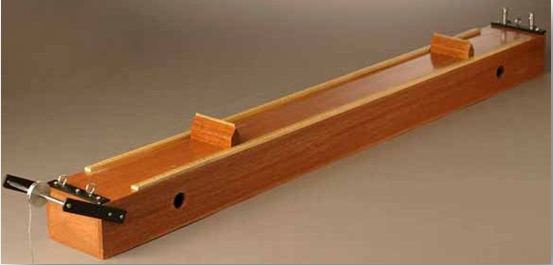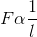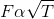you are here->home->Physics->Class 11->Sonometer
Sonometer

# Objective

• To study the relation between frequency and length of a given wire under constant tension using sonometer. To plot a graph between ν and l.
• To study the relation between length of a given wire and tension for constant frequency using sonometer. To plot a graph between l2 and T.

# Theory

## What is a Sonometer ?

Sonometer consists of a hollow rectangular wooden box of more than one meter length, with a hook at one end and a pulley at the other end. One end of a string is fixed at the hook and the other end passes over the pulley. A weight hanger is attached to the free end of the string. Two adjustable wooden bridges are put over the board, so that the length of string can be adjusted.## Production of transverse waves in stretched strings

If a string which is stretched between two fixed points is plucked at its center, vibrations produced and it move out in opposite directions along the string. Because of this, a transverse wave travels along the string.

If a string of length l having mass per unit length m is stretched with a tension T, the fundamental frequency of vibration f is given by;## Laws of transverse vibrations on a stretched string

• Law of Length: The frequency of vibration of a stretched string varies inversely as its resonating length (provided its mass per unit length and tension remain constant.)• Law of Tension:The frequency of vibration of a stretched string varies directly as the square root of its tension, (provided its resonating length and mass per unit length of the wire remains constant).### Relation between frequency and length

From the law of length,  f ×l = constant

A graph between f and 1/l will be a straight line.

### Relation between length and tension

From the equation for frequency, √T / l = constant

A graph between T and l2 will be a straight line.

# Learning outcomes

• Students develop the idea about standing waves.
• Students understand the sonometer apparatus and its working.
• Students get the relation between frequency, length and tension of a stretched string under vibration.